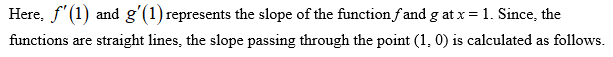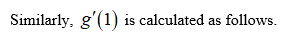# -1

Question
22 views

The graphs of the function f (given in blue) and g(given in red) are plotted above. Suppose that u(x) = f(x)g(x) and v(x)=f(x)/g(x). Find each of the following:

u′(1) = ?

v′(1) = ?

check_circle

Step 1

From the given graph it can clearly observed that f (1) = 1 and g (1) = 3.

Given that u (x) = f (x) g (x), then

Step 2Step 3...

### Want to see the full answer?

See Solution

#### Want to see this answer and more?

Solutions are written by subject experts who are available 24/7. Questions are typically answered within 1 hour.*

See Solution
*Response times may vary by subject and question.
Tagged in

### Derivative